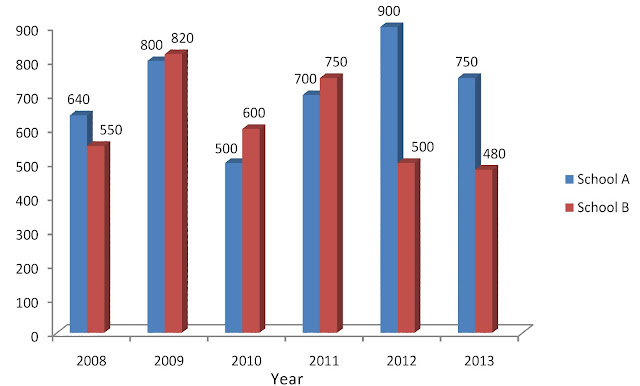New Students Offer - Use Code HELLO

# Solve Bar Graph By Visual Inspection

In this article we learn how we can calculate BAR GRAPH easily by solving most repeatedly asked questions in our examinations.

#### Guidelines:

1. Whenever we solve DI questions first we need to analyse the given data to proceed further.
2. Observe what is on x-axis and y-axis, Observe the colour for which it is specified.

Directions: The following bar graph shows the total number of students appearing in an examination from two schools A and B over the years.Ques.  What is the average number of students appearing from School A over the given period?
A. 705
B. 710
C. 715
D. 720
E. 725

#### TRICK 1:

Observe the bar graph to find average never take a note of it and calculate. Calculate in your mind.
Explanation: 640 800 500 700 900 750
Take 700 as base (for example)
-60 +100 -200 0 +200 +50 =+90/6=15

#### TRICK 2:

Turn every year value to base value to perform simpler calculation
Take 700(2011) as base
Give 200(from 900-200=700) (2012) to (2010) 500+200=700
Give 50(from 750-50=700) (2013) to (2008) 640+50=690
Give 10(from 800-10=790) (2009) to (2008) 690+10=700
Left over is 90/6=15